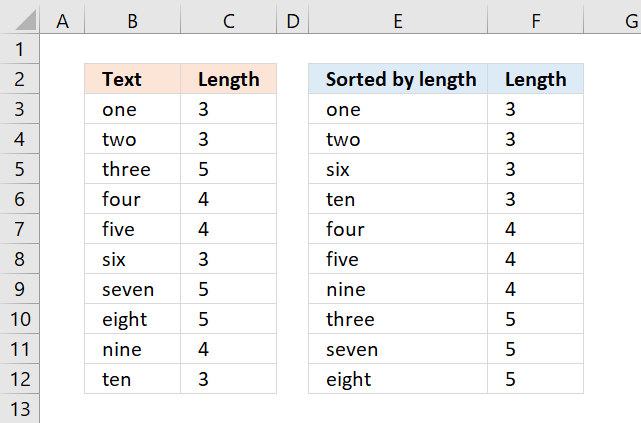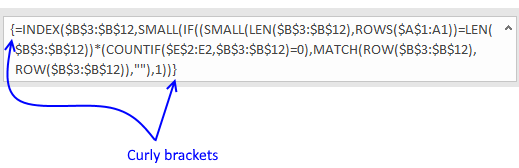Author: Oscar Cronquist Article last updated on July 09, 2022This article demonstrates a formula that sorts text values based on character length, the Excel 365 dynamic array formula is considerably smaller and is a great example of how amazing the new Excel 365 functions are.

The image above shows the result in cell E3 and cells below, the formula is returning values from cell range B3:B12 from small to large (ascending order). Cell range C3:C12 shows the string length of the corresponding cell on the same row.

## 1. SMALL function for textThe array formula in column E, shown in above picture sorts text values from column B. The Length columns prove that they are sorted by text length from small to large. Don't use this formula if you are an Excel 365 subscriber, read section 2.

=INDEX(\$B\$3:\$B\$12, SMALL(IF((SMALL(LEN(\$B\$3:\$B\$12), ROWS(\$A\$1:A1))=LEN(\$B\$3:\$B\$12))*(COUNTIF(\$E\$2:E2, \$B\$3:\$B\$12)=0), MATCH(ROW(\$B\$3:\$B\$12), ROW(\$B\$3:\$B\$12)), ""), 1))

### 1.1 How to enter an array formula

To enter an array formula, type the formula in a cell then press and hold CTRL + SHIFT simultaneously, now press Enter once. Release all keys.The formula bar now shows the formula with a beginning and ending curly bracket telling you that you entered the formula successfully. Don't enter the curly brackets yourself.

### 1.2 Explaining formula

#### Step 1 - Calculate string length

The LEN function returns a number representing the number of characters in a given cell.

LEN(text)

LEN(\$B\$3:\$B\$12)

becomes

LEN({"one"; "two"; "three"; "four"; "five"; "six"; "seven"; "eight"; "nine"; "ten"})

and returns

{3; 3; 5; 4; 4; 3; 5; 5; 4; 3}.

#### Step 2 - Extract the k-th smallest number

The SMALL function returns the k-th smallest value from a group of numbers.

SMALL(array, k)

SMALL(LEN(\$B\$3:\$B\$12), ROWS(\$A\$1:A1))

becomes

SMALL({3; 3; 5; 4; 4; 3; 5; 5; 4; 3}, ROWS(\$A\$1:A1))

The ROWS function allows you to calculate the number of rows in a cell range.

ROWS(array)

The cell reference \$A\$1:A1 has two parts, an absolute and a relative part. When you copy the cell and paste it to the cells below the relative part changes accordingly. This will calculate a new number in each cell, the first cell evaluates to 1, the next cell below evaluates to two, and so on.

SMALL({3; 3; 5; 4; 4; 3; 5; 5; 4; 3}, ROWS(\$A\$1:A1))

becomes

SMALL({3; 3; 5; 4; 4; 3; 5; 5; 4; 3}, 1)

and returns 3.

#### Step 3 - Compare the result to the array

The equal sign is a logical operator, it allows you to compare value to value or in this case value to an array. The result is a boolean value, TRUE or FALSE.

SMALL(LEN(\$B\$3:\$B\$12), ROWS(\$A\$1:A1))=LEN(\$B\$3:\$B\$12)

becomes

3=LEN(\$B\$3:\$B\$12)

becomes

3={3; 3; 5; 4; 4; 3; 5; 5; 4; 3}

and returns

{TRUE; TRUE; FALSE; FALSE; FALSE; TRUE; FALSE; FALSE; FALSE; TRUE}.

#### Step 4 - Count previous results above and compare result with zero

The COUNTIF function calculates the number of cells that is equal to a condition.

COUNTIF(rangecriteria)

(COUNTIF(\$E\$2:E2, \$B\$3:\$B\$12)=0

becomes

{0;0;0;0;0;0;0;0;0;0}=0

and returns

{TRUE; TRUE; TRUE; TRUE; TRUE; TRUE; TRUE; TRUE; TRUE; TRUE}.

This means that there have not been displayed any values above yet.

#### Step 5 - Multiply arrays

We will now multiply the array containing character length with the array containing where the k-th smallest number is.

(SMALL(LEN(\$B\$3:\$B\$12), ROWS(\$A\$1:A1))=LEN(\$B\$3:\$B\$12))*(COUNTIF(\$E\$2:E2, \$B\$3:\$B\$12)=0)

becomes

{TRUE; TRUE; FALSE; FALSE; FALSE; TRUE; FALSE; FALSE; FALSE; TRUE}*(COUNTIF(\$E\$2:E2, \$B\$3:\$B\$12)=0)

becomes

{TRUE; TRUE; FALSE; FALSE; FALSE; TRUE; FALSE; FALSE; FALSE; TRUE}*{TRUE; TRUE; TRUE; TRUE; TRUE; TRUE; TRUE; TRUE; TRUE; TRUE}

and returns

{1; 1; 0; 0; 0; 1; 0; 0; 0; 1}.

When you perform a calculation to boolean values the result is always the numerical equivalent.

TRUE = 1 and FALSE = 0 (zero)

#### Step 6 - Replace TRUE with corresponding row number

The IF function returns one value if the logical test is TRUE and another value if the logical test is FALSE.

IF(logical_test, [value_if_true], [value_if_false])

IF((SMALL(LEN(\$B\$3:\$B\$12), ROWS(\$A\$1:A1))=LEN(\$B\$3:\$B\$12))*(COUNTIF(\$E\$2:E2, \$B\$3:\$B\$12)=0), MATCH(ROW(\$B\$3:\$B\$12), ROW(\$B\$3:\$B\$12)), "")

becomes

IF({1; 1; 0; 0; 0; 1; 0; 0; 0; 1}, MATCH(ROW(\$B\$3:\$B\$12), ROW(\$B\$3:\$B\$12)), "")

The ROW and MATCH function combined creates a sequence of numbers from 1 to n where n is the number of rows in \$B\$3:\$B\$12

MATCH(ROW(\$B\$3:\$B\$12), ROW(\$B\$3:\$B\$12))

becomes

MATCH({3;4;5;6;7;8;9;10;11;12}, {3;4;5;6;7;8;9;10;11;12})

and returns

{1; 2; 3; 4; 5; 6; 7; 8; 9; 10}.

IF({1; 1; 0; 0; 0; 1; 0; 0; 0; 1}, MATCH(ROW(\$B\$3:\$B\$12), ROW(\$B\$3:\$B\$12)), "")

becomes

IF({1; 1; 0; 0; 0; 1; 0; 0; 0; 1}, {1; 2; 3; 4; 5; 6; 7; 8; 9; 10}, "")

and returns

{1; 2; ""; ""; ""; 6; ""; ""; ""; 10}.

#### Step 7 - Extract the smallest row number

SMALL(IF((SMALL(LEN(\$B\$3:\$B\$12), ROWS(\$A\$1:A1))=LEN(\$B\$3:\$B\$12))*(COUNTIF(\$E\$2:E2, \$B\$3:\$B\$12)=0), MATCH(ROW(\$B\$3:\$B\$12), ROW(\$B\$3:\$B\$12)), ""), 1)

becomes

SMALL({1; 2; ""; ""; ""; 6; ""; ""; ""; 10}, 1)

and returns 1.

#### Step 8 - Get value

The INDEX function returns a value from a cell range, you specify which value based on a row and column number.

INDEX(\$B\$3:\$B\$12, SMALL(IF((SMALL(LEN(\$B\$3:\$B\$12), ROWS(\$A\$1:A1))=LEN(\$B\$3:\$B\$12))*(COUNTIF(\$E\$2:E2, \$B\$3:\$B\$12)=0), MATCH(ROW(\$B\$3:\$B\$12), ROW(\$B\$3:\$B\$12)), ""), 1))

becomes

INDEX(\$B\$3:\$B\$12, 1)

and returns "one" in cell F3.

If you are looking for a formula that sorts text alphabetically, check this out: Sort a column alphabetically

## 2. SMALL function for text - Excel 365The formula in cell E3 sorts the text values in cell range B3:B12 based on character length from small to large.

Dynamic array formula in cell E3:

=SORTBY(B3:B12,LEN(B3:B12),1)

### 2.1 How to enter a dynamic array formula

The formula in cell E3 works only in Excel 365, it is entered as a regular formula however, it spills to cells below as far as needed.

### 2.2 Explaining formula

#### Step 1 - Calculate string length

The LEN function returns a number representing the number of characters in a given cell.

LEN(text)

LEN(B3:B12)

becomes

LEN({"one"; "two"; "three"; "four"; "five"; "six"; "seven"; "eight"; "nine"; "ten"})

and returns

{3; 3; 5; 4; 4; 3; 5; 5; 4; 3}.

#### Step 2 - Sort by string length

The SORTBY function allows you to sort values from a cell range or array based on a corresponding cell range or array. It sorts values by column but keeps rows.

SORTBY(array, by_array1, [sort_order1], [by_array2, sort_order2],…)

SORTBY(B3:B12, LEN(B3:B12), 1)

becomes

SORTBY(B3:B12, {3; 3; 5; 4; 4; 3; 5; 5; 4; 3}, 1)

and returns

{"one"; "two"; "six"; "ten"; "four"; "five"; "nine"; "three"; "seven"; "eight"}.